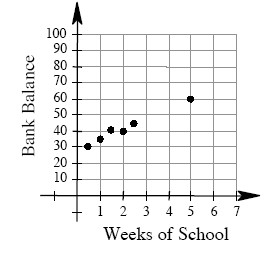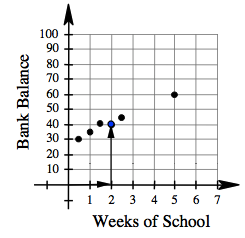### Home > AC > Chapter 2 > Lesson 2.2.1 > Problem2-98

2-98.

Since the beginning of school, Steven has been saving money to buy a new MP3 player. His bank balance is represented by the graph below.1. According to the graph, about how much money had Steven saved after $2$ weeks of school?He saved $\40$.

2. About how much money did Steven probably have after $4$ weeks of school? How can you tell?

Imagine a line that best represents the trend of the graph.

What is the $y$-coordinate of the line when $x$ equals $4$?

$\approx\55$

3. If he keeps saving at the same rate, how much will he have saved by Week $7$? Explain how you know.

Follow the steps in part (b).